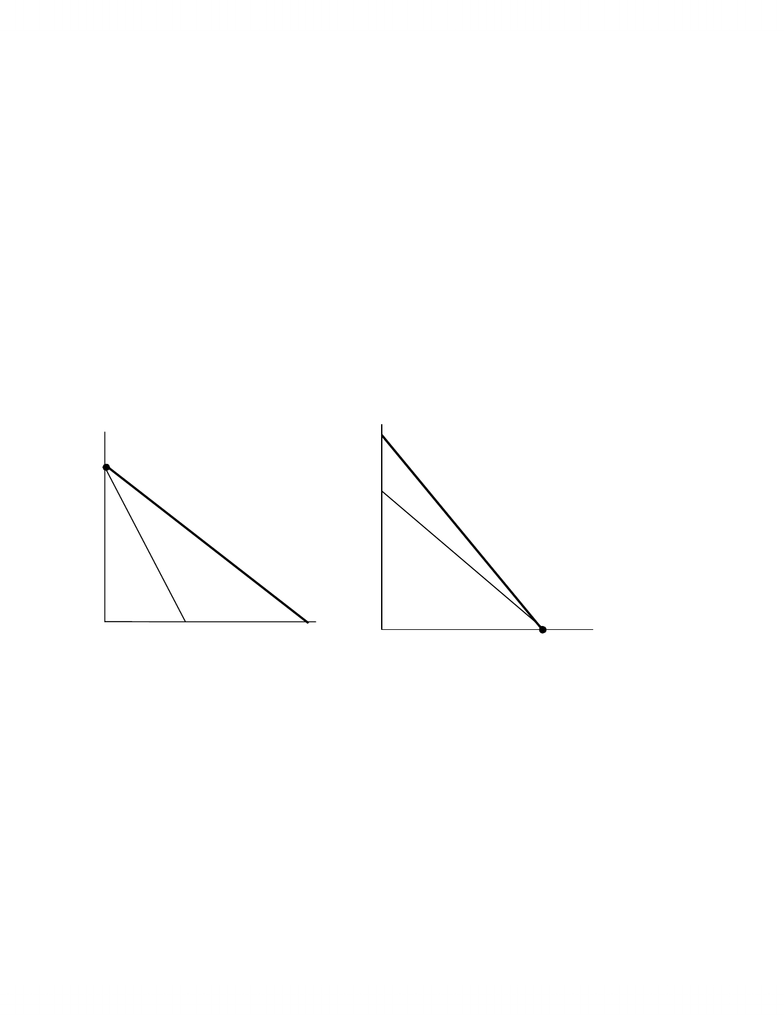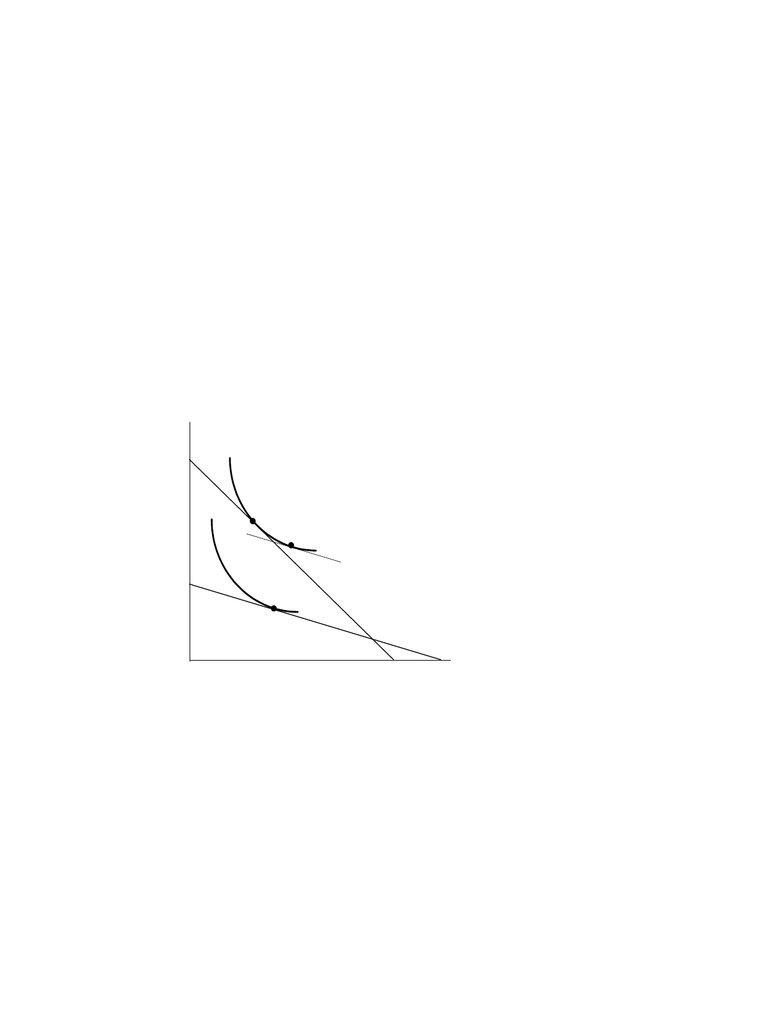# ECO101H1 Chapter Notes - Chapter 4: European Route E40, Isocost, Isoquant

34 views7 pages
School
UTSG
Department
Economics
Course
ECO101H122
CHAPTER 4
4-1. Suppose there are two inputs in the production function, labor and capital, and these two
inputs are perfect substitutes. The existing technology permits 1 machine to do the work of 3
persons. The firm wants to produce 100 units of output. Suppose the price of capital is \$750 per
machine per week. What combination of inputs will the firm use if the weekly salary of each worker
is \$300? What combination of inputs will the firm use if the weekly salary of each worker is \$225?
What is the elasticity of labor demand as the wage falls from \$300 to \$225?
Because labor and capital are perfect substitutes, the isoquants (in bold) are linear and the firm will use
only labor or only capital, depending on which is cheaper in producing 100 units of output.
The (absolute value of the) slope of the isoquant (MPE / MPK) is 1/3 because 1 machine does the work of
3 men. When the wage is \$900 (left panel), the slope of the isocost is 300/750. The isocost curve,
therefore, is steeper than the isoquant, and the firm only hires capital (at point A). When the weekly wage
is \$225 (right panel), the isoquant is steeper than the isocost and the firm hires only labor (at point B).
Weekly Salary = \$300 Weekly Salary = \$225
The elasticity of labor demand is defined as the percentage change in labor divided by the percentage
change in the wage. Because the demand for labor goes from 0 to a positive quantity when the wage
dropped to \$225, the (absolute value of the) elasticity of labor demand is infinity.
Labor
Capital
slope = w/r
=300/750
slope=MPE /MPK
=1/3
A
Labor
Capital
slope = w/r
=225/750
slope =MPE /MPK
=1/3
B
Unlock document

This preview shows pages 1-2 of the document.
Unlock all 7 pages and 3 million more documents.23
4-2. (a) What happens to the long-run demand curve for labor if the demand for the firm’s output
increases?
The labor demand curve is given by VMPE = MR x MPE. As demand for the firm’s output increases, its
marginal revenue also increases. Thus, an increase in demand for the firm’s output shifts the labor
demand curve to the right.
(b) What happens to the long-run demand curve for labor if the price of capital increases?
To determine how an increase in the price of capital changes the demand for labor, suppose initially that
the firm is producing 200 units of output at point P in the figure. The increase in the price of capital
(assuming capital is a normal input) increases the marginal costs of the firm and will reduce the profit-
maximizing level of output to say 100 units. The increase in the price of capital also flattens the isocost
curve, moving the firm to point R. The move from point P to point R can be decomposed into a
substitution effect (P to Q) which reduces the demand for capital, but increases the demand for labor, and
a scale effect (Q to R) which reduces the demand for both labor and capital. The direction of the shift in
the demand curve for labor, therefore, will depend on which effect is stronger: the scale effect or the
substitution effect.
100
200
R
P
Q
Capital
Employment
Unlock document

This preview shows pages 1-2 of the document.
Unlock all 7 pages and 3 million more documents.